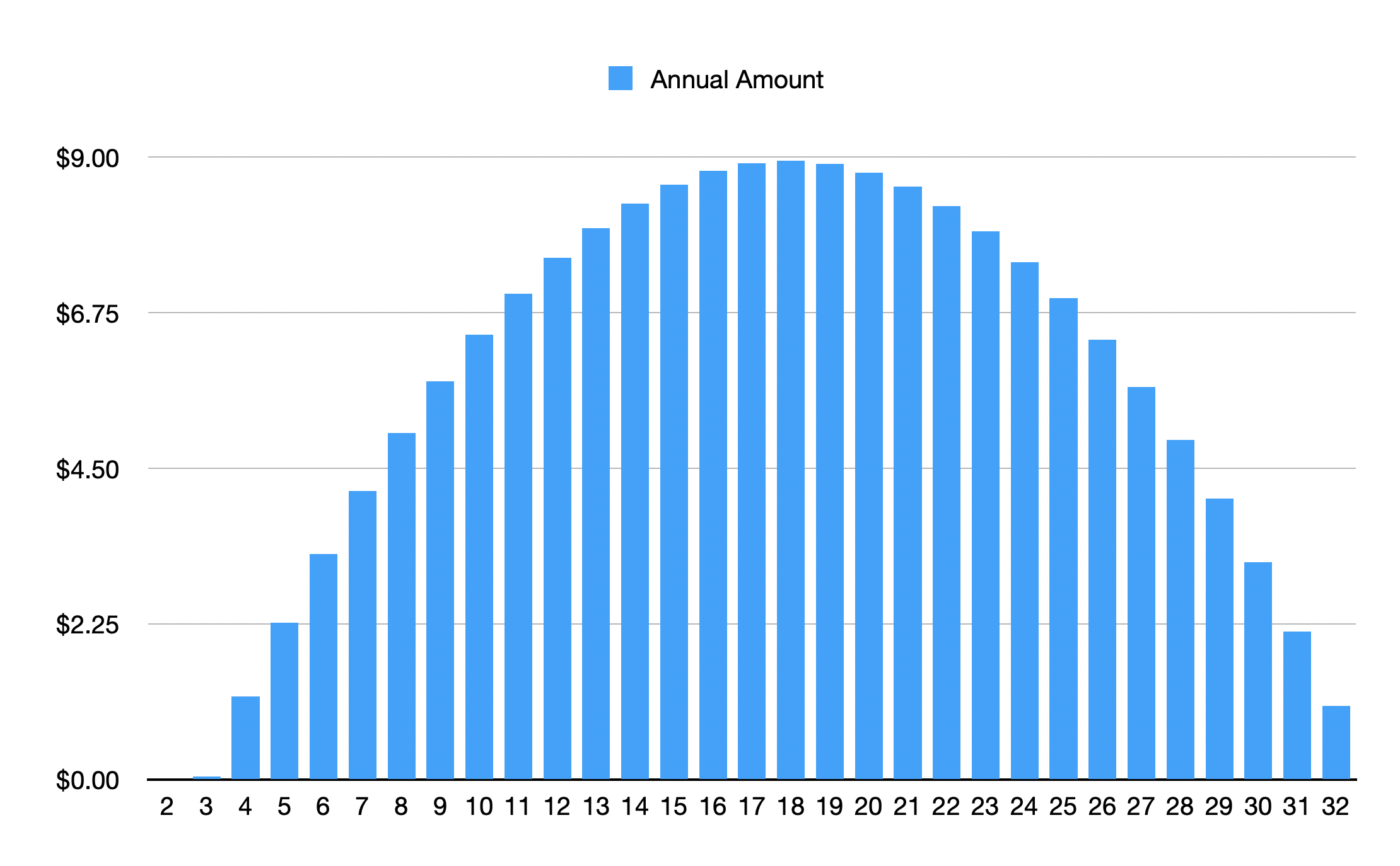Start a new topic

## Calculating Net Revenue over time

For the Carbon Tax, I want to calculate the Net Revenue over time.
If I use the Graph:  Revenue & Cost from Taxes & Subsidies.
Download the data into a spreadsheet, plot the data on a graph and fit the curve to a polynomial, then I should get the same graph as En-Roads, and it looks the same to me.

If I integrate and find the area under the curve for five years, and ten years, then I would know the total net revenue from a carbon tax for those two periods.

Using my scenario, Renewables+CarbonPrice+AssumptionChanges+EnergyEfficiency+Electrification+Methane

I calculate \$7.78 Trillion over five years and \$25.1 Trillion over ten years.
This would then the be the money available to global governments to invest for equity for those hit hardest by increase in energy prices, and for equity and jobs, and healthcare.

Did I do that right?

correction to my first post.

put everything into a spreadsheet so that when I found errors I could quickly recalculate.....

Draft:

See attached PDF

My calculus chops are not what they were, but it seems right. Note that these are annual revenue (trillion \$/year) reported annually - so the simple sum is very close to the cumulative value - picture a bar graph compared to your fitted curve; the area of each bar is revenue per year times one year. That is probably close enough if you need to do it on the fly, and intuitive to an audience.

Yes, like this below.  polynomial curve fit approximated by series of bars.Corrected the x-axis label from 2-32, should have been 0-30, from 2020-2030.

Here is the graph of cumulative net revenue over 30 years.

Looks like an "S" curve, and based on the shape of the annual amount.You should not have to do that step - the series of bars approximating the polynomial you estimated from a series of numbers. Those bars are (if you space by one year) a way of estimating the numbers you started with. You can just use the En-ROADS output directly - as an approximation, it is not as good as your fitted polynomial integration, but good enough.

Note that the underlying model uses numerical approximations for every integration or accumulation in the system. We use an eighth-year time step, but otherwise, adding the output is the same way we would calculate in the software if we had a cumulative net revenue graph

Yes, some of my calculations and graph are repetitive steps.

Thanks for confirming that I'm on the right track with these calculations.